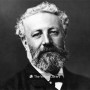# Eight hundred leagues on The Amazon

Book ID: 306
Sometimes it is not possible to find the cover corresponding to the book whose edition is published. Please, consider this image only as a reference, it will not always be the exact cover used in the edition of the published book.
Created by## Jules Verne / Julio Verne (83 books)

Wikipedia:

A CAPTAIN OF THE WOODS

“P h y j s l y d d q f d z x g a s g z z q q e h x g k f n d r x u j u g I o c y t d x v k s b x h h u y p o h d v y r y m h u h p u y d k j o x p h e t o z l s l e t n p m v f f o v p d p a j x h y y n o j y g g a y m e q y n f u q l n m v l y f g s u z m q I z t l b q q y u g s q e u b v n r c r e d g r u z b l r m x y u h q h p z d r r g c r o h e p q x u f I v v r p l p h o n t h v d d q f h q s n t z h h h n f e p m q k y u u e x k t o g z g k y u u m f v I j d q d p z j q s y k r p l x h x q r y m v k l o h h h o t o z v d k s p p s u v j h d.”

The man who held in his hand the document of which this strange assemblage of letters formed the concluding paragraph remained for some moments lost in thought.

It contained about a hundred of these lines, with the letters at even distances, and undivided into words. It seemed to have been written many years before, and time had already laid his tawny finger on the sheet of good stout paper which was covered with the hieroglyphics.

On what principle had these letters been arranged? He who held the paper was alone able to tell. With such cipher language it is as with the locks of some of our iron safes—in either case the protection is the same. The combinations which they lead to can be counted by millions, and no calculator’s life would suffice to express them. Some particular “word” has to be known before the lock of the safe will act, and some “cipher” is necessary before that cryptogram can be read.[...]

Jules Verne - Julio Verne - Жюль Верн - جول فيرن

Jules Verne · English

####Public Domain content

Except where otherwise noted, all the contents published in this website are in the Public Domain. This includes original texts, translations and book covers. You can share and adapt it for any use. Please, refer to About Us section for further information.